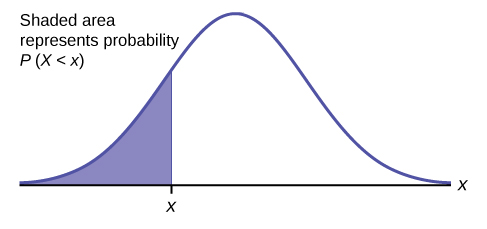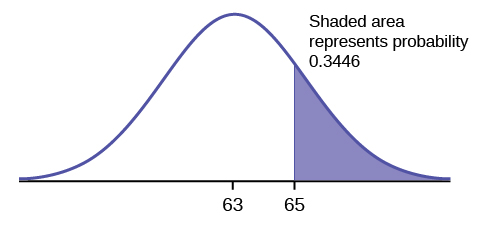# 3.3: Using the Normal Distribution

The shaded area in the following graph indicates the area to the left of $$x$$. This area is represented by the probability $$P(X < x)$$. Normal tables, computers, and calculators provide or calculate the probability $$P(X < x)$$.Figure $$\PageIndex{1}$$.

The area to the right is then $$P(X > x) = 1 – P(X < x)$$. Remember, $$P(X < x) =$$ Area to the left of the vertical line through $$x$$. $$P(X < x) = 1 – P(X < x) =$$ Area to the right of the vertical line through $$x$$. $$P(X < x)$$ is the same as $$P(X \leq x)$$ and $$P(X > x)$$ is the same as $$P(X \geq x)$$ for continuous distributions.

## Calculations of Probabilities

Probabilities are calculated using technology. There are instructions given as necessary for the TI-83+ and TI-84 calculators.To calculate the probability, use the probability tables provided in [link] without the use of technology. The tables include instructions for how to use them.

Example $$\PageIndex{1}$$

If the area to the left is 0.0228, then the area to the right is $$1 - 0.0228 = 0.9772$$.

Exercise $$\PageIndex{1}$$

If the area to the left of $$x$$ is $$0.012$$, then what is the area to the right?

$$1 - 0.012 = 0.988$$

Example $$\PageIndex{2}$$

The final exam scores in a statistics class were normally distributed with a mean of 63 and a standard deviation of five.

1. Find the probability that a randomly selected student scored more than 65 on the exam.
2. Find the probability that a randomly selected student scored less than 85.
3. Find the 90th percentile (that is, find the score $$k$$ that has 90% of the scores below k and 10% of the scores above $$k$$).
4. Find the 70th percentile (that is, find the score $$k$$ such that 70% of scores are below $$k$$ and 30% of the scores are above $$k$$).

a. Let $$X$$ = a score on the final exam. $$X \sim N(63, 5)$$, where $$\mu = 63$$ and $$\sigma = 5$$

Draw a graph.

Then, find $$P(x > 65)$$.

$P(x > 65) = 0.3446\nonumber$Figure $$\PageIndex{2}$$.

The probability that any student selected at random scores more than 65 is 0.3446.

Go into 2nd DISTR.

After pressing 2nd DISTR, press 2:normalcdf.

The syntax for the instructions are as follows:

normalcdf(lower value, upper value, mean, standard deviation) For this problem: normalcdf(65,1E99,63,5) = 0.3446. You get 1E99 (= 1099) by pressing 1, the EE key (a 2nd key) and then 99. Or, you can enter 10^99instead. The number 1099 is way out in the right tail of the normal curve. We are calculating the area between 65 and 1099. In some instances, the lower number of the area might be –1E99 (= –1099). The number –1099 is way out in the left tail of the normal curve.

Historical Note

The TI probability program calculates a $$z$$-score and then the probability from the $$z$$-score. Before technology, the $$z$$-score was looked up in a standard normal probability table (because the math involved is too cumbersome) to find the probability. In this example, a standard normal table with area to the left of the $$z$$-score was used. You calculate the $$z$$-score and look up the area to the left. The probability is the area to the right.

$z = 65 – 63565 – 635 = 0.4\nonumber$

Area to the left is 0.6554.

$P(x > 65) = P(z > 0.4) = 1 – 0.6554 = 0.3446\nonumber$

Calculate the $$z$$-score:

*Press 2nd Distr

*Press 3:invNorm(*Enter the area to the left of $$z$$ followed by)
*Press ENTER.
For this Example, the steps are
2nd Distr
3:invNorm(.6554) ENTER

The answer is 0.3999 which rounds to 0.4.

b. Draw a graph.

Then find $$P(x < 85)$$, and shade the graph.

Using a computer or calculator, find $$P(x < 85) = 1$$.

$$\text{normalcdf}(0,85,63,5) = 1$$ (rounds to one)

The probability that one student scores less than 85 is approximately one (or 100%).

c. Find the 90th percentile. For each problem or part of a problem, draw a new graph. Draw the $$x$$-axis. Shade the area that corresponds to the 90th percentile.

Let $$k =$$ the 90th percentile. The variable $$k$$ is located on the $$x$$-axis. $$P(x < k)$$ is the area to the left of $$k$$. The 90th percentile $$k$$ separates the exam scores into those that are the same or lower than $$k$$ and those that are the same or higher. Ninety percent of the test scores are the same or lower than $$k$$, and ten percent are the same or higher. The variable $$k$$ is often called a critical value.

$$k = 69.4$$Figure $$\PageIndex{3}$$.

The 90th percentile is 69.4. This means that 90% of the test scores fall at or below 69.4 and 10% fall at or above. To get this answer on the calculator, follow this step:

invNorm in 2nd DISTR. invNorm(area to the left, mean, standard deviation)

For this problem, $$\text{invNorm}(0.90,63,5) = 69.4$$

d. Find the 70th percentile.

Draw a new graph and label it appropriately. $$k = 65.6$$

The 70th percentile is 65.6. This means that 70% of the test scores fall at or below 65.5 and 30% fall at or above.

$$\text{invNorm}(0.70,63,5) = 65.6$$

Exercise $$\PageIndex{2}$$

The golf scores for a school team were normally distributed with a mean of 68 and a standard deviation of three. Find the probability that a randomly selected golfer scored less than 65.

$$\text{normalcdf}(10^{99},65,68,3) = 0.1587$$

Example $$\PageIndex{3}$$

A personal computer is used for office work at home, research, communication, personal finances, education, entertainment, social networking, and a myriad of other things. Suppose that the average number of hours a household personal computer is used for entertainment is two hours per day. Assume the times for entertainment are normally distributed and the standard deviation for the times is half an hour.

1. Find the probability that a household personal computer is used for entertainment between 1.8 and 2.75 hours per day.
2. Find the maximum number of hours per day that the bottom quartile of households uses a personal computer for entertainment.

a. Let $$X =$$ the amount of time (in hours) a household personal computer is used for entertainment. $$X \sim N(2, 0.5)$$ where $$\mu = 2$$ and $$\sigma = 0.5$$.

Find $$P(1.8 < x < 2.75)$$.

The probability for which you are looking is the area between $$x = 1.8$$ and $$x = 2.75$$. $$P(1.8 < x < 2.75) = 0.5886$$Figure $$\PageIndex{4}$$.

$\text{normalcdf}(1.8,2.75,2,0.5) = 0.5886\nonumber$

The probability that a household personal computer is used between 1.8 and 2.75 hours per day for entertainment is 0.5886.

b.

To find the maximum number of hours per day that the bottom quartile of households uses a personal computer for entertainment, find the 25th percentile, $$k$$, where $$P(x < k) = 0.25$$.Figure $$\PageIndex{5}$$.

$\text{invNorm}(0.25,2,0.5) = 1.66\nonumber$

The maximum number of hours per day that the bottom quartile of households uses a personal computer for entertainment is 1.66 hours.

Exercise $$\PageIndex{3}$$

The golf scores for a school team were normally distributed with a mean of 68 and a standard deviation of three. Find the probability that a golfer scored between 66 and 70.

$$\text{normalcdf}(66,70,68,3) = 0.4950$$

Example $$\PageIndex{4}$$

There are approximately one billion smartphone users in the world today. In the United States the ages 13 to 55+ of smartphone users approximately follow a normal distribution with approximate mean and standard deviation of 36.9 years and 13.9 years, respectively.

1. Determine the probability that a random smartphone user in the age range 13 to 55+ is between 23 and 64.7 years old.
2. Determine the probability that a randomly selected smartphone user in the age range 13 to 55+ is at most 50.8 years old.
3. Find the 80th percentile of this distribution, and interpret it in a complete sentence.

a. $$\text{normalcdf}(23,64.7,36.9,13.9) = 0.8186$$

b. $$\text{normalcdf}(-10^{99},50.8,36.9,13.9) = 0.8413$$

c. $$\text{invNorm}(0.80,36.9,13.9) = 48.6$$

The 80th percentile is 48.6 years.

80% of the smartphone users in the age range 13 – 55+ are 48.6 years old or less.

Use the information in Example to answer the following questions.

Exercise $$\PageIndex{4}$$

1. Find the 30th percentile, and interpret it in a complete sentence.
2. What is the probability that the age of a randomly selected smartphone user in the range 13 to 55+ is less than 27 years old.

70.

Let $$X =$$ a smart phone user whose age is 13 to 55+. $$X \sim N(36.9, 13.9)$$

To find the 30th percentile, find $$k$$ such that $$P(x < k) = 0.30$$.
$$\text{invNorm}(0.30, 36.9, 13.9) = 29.6$$ years
Thirty percent of smartphone users 13 to 55+ are at most 29.6 years and 70% are at least 29.6 years. Find $$P(x < 27)$$
(Note that $$\text{normalcdf}(-10^{99},27,36.9,13.9) = 0.2382$$. The two answers differ only by 0.0040.)Figure $$\PageIndex{6}$$.

$\text{normalcdf}(0,27,36.9,13.9) = 0.2342\nonumber$

Example $$\PageIndex{5}$$

There are approximately one billion smartphone users in the world today. In the United States the ages 13 to 55+ of smartphone users approximately follow a normal distribution with approximate mean and standard deviation of 36.9 years and 13.9 years respectively. Using this information, answer the following questions (round answers to one decimal place).

1. Calculate the interquartile range ($$IQR$$).
2. Forty percent of the ages that range from 13 to 55+ are at least what age?

a.

$IQR = Q_{3} – Q_{1}\nonumber$

Calculate $$Q_{3} =$$ 75th percentile and $$Q_{1} =$$ 25th percentile.

$\text{invNorm}(0.75,36.9,13.9) = Q_{3} = 46.2754\nonumber$

$\text{invNorm}(0.25,36.9,13.9) = Q_{1} = 27.5246\nonumber$

$IQR = Q_{3} - Q_{1} = 18.7508\nonumber$

b.

Find $$k$$ where $$P(x > k) = 0.40$$ ("At least" translates to "greater than or equal to.")

$$0.40 =$$ the area to the right.

Area to the left $$= 1 – 0.40 = 0.60$$.

The area to the left of $$k = 0.60$$.

$$\text{invNorm}(0.60,36.9,13.9) = 40.4215$$.

$$k = 40.42$$.

Forty percent of the ages that range from 13 to 55+ are at least 40.42 years.

Exercise $$\PageIndex{5}$$

Two thousand students took an exam. The scores on the exam have an approximate normal distribution with a mean $$\mu = 81$$ points and standard deviation $$\sigma = 15$$ points.

1. Calculate the first- and third-quartile scores for this exam.
2. The middle 50% of the exam scores are between what two values?

1. $$Q_{1} =$$ 25th percentile $$= \text{invNorm}(0.25,81,15) = 70.9$$
$$Q_{3} =$$ 75th percentile $$= \text{invNorm}(0.75,81,15) = 91.9$$
2. The middle 50% of the scores are between 70.9 and 91.1.

Example $$\PageIndex{6}$$

A citrus farmer who grows mandarin oranges finds that the diameters of mandarin oranges harvested on his farm follow a normal distribution with a mean diameter of 5.85 cm and a standard deviation of 0.24 cm.

1. Find the probability that a randomly selected mandarin orange from this farm has a diameter larger than 6.0 cm. Sketch the graph.
2. The middle 20% of mandarin oranges from this farm have diameters between ______ and ______.
3. Find the 90th percentile for the diameters of mandarin oranges, and interpret it in a complete sentence.

a. $$\text{normalcdf}(6,10^{99},5.85,0.24) = 0.2660$$Figure $$\PageIndex{7}$$.

b.

$$1 – 0.20 = 0.80$$

The tails of the graph of the normal distribution each have an area of 0.40.

Find $$k1$$, the 40th percentile, and $$k2$$, the 60th percentile ($$0.40 + 0.20 = 0.60$$).

$$k1 = \text{invNorm}(0.40,5.85,0.24) = 5.79$$ cm

$$k2 = \text{invNorm}(0.60,5.85,0.24) = 5.91$$ cm

c. 6.16: Ninety percent of the diameter of the mandarin oranges is at most 6.15 cm.

Exercise $$\PageIndex{6}$$

Using the information from Example, answer the following:

1. The middle 45% of mandarin oranges from this farm are between ______ and ______.
2. Find the 16th percentile and interpret it in a complete sentence.

The middle area $$= 0.40$$, so each tail has an area of 0.30.

$$– 0.40 = 0.60$$

The tails of the graph of the normal distribution each have an area of 0.30.

Find $$k1$$, the 30th percentile and $$k2$$, the 70th percentile ($$0.40 + 0.30 = 0.70$$).

$$k1 = \text{invNorm}(0.30,5.85,0.24) = 5.72$$ cm

$$k2 = \text{invNorm}(0.70,5.85,0.24) = 5.98$$ cm

$$\text{normalcdf}(5,10^{99},5.85,0.24) = 0.9998$$